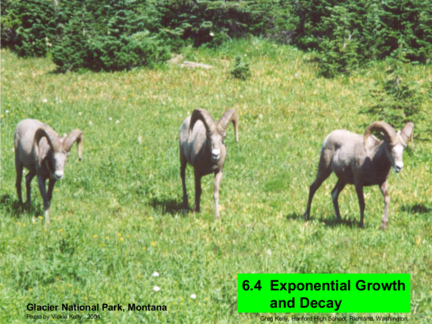# How to Calculate Exponential Growth and Decay using CalculusContributed by:We will be discussing the exponential rates while working on integrals. We will also see the topics: radioactive decay, half-life, and Newton's Law of cooling.
1. 6.4 Exponential Growth
Glacier National Park, Montana and Decay
Photo by Vickie Kelly, 2004 Greg Kelly, Hanford High School, Richland, Washington
2. The number of bighorn sheep in a population increases at
a rate that is proportional to the number of sheep present
(at least for awhile.)
So does any population of living creatures. Other things
that increase or decrease at a rate proportional to the
amount present include radioactive material and money in
an interest-bearing account.
If the rate of change is proportional to the amount present,
the change can be modeled by:
dy
ky
dt

3. dy Rate of change is proportional
ky
dt to the amount present.
dy k dt Divide both sides by y.
1
y dy k dt Integrate both sides.
ln y kt  C

4. 1
y dy k dt Integrate both sides.
ln y kt  C
ln y kt C Exponentiate both sides.
e e
C kt When multiplying like bases, add
y e e exponents. So added exponents
can be written as multiplication.

5. ln y kt C
Exponentiate both sides.
e e
C kt When multiplying like bases, add
y e e exponents. So added exponents
can be written as multiplication.
C kt
y e e
kt
y  Ae Since eC is a constant, let e
C
A .

6. C kt
y e e
kt
y  Ae Since eC is a constant, let e
C
A .
1
k 0
y0  Ae At t 0 , y  y0 .
y0  A
kt This is the solution to our original initial
y  y0 e value problem.

7. kt
Exponential Change: y  y0 e
If the constant k is positive then the equation
represents growth. If k is negative then the equation
represents decay.
Note: This lecture will talk about exponential change
formulas and where they come from. The problems in
this section of the book mostly involve using those
formulas. There are good examples in the book, which I
will not repeat here.

8. Continuously Compounded Interest
If money is invested in a fixed-interest account where the
interest is added to the account k times per year, the
amount present after t years is:
kt
 r
A  t   A0  1  
 k
If the money is added back more frequently, you will make
a little more money.
The best you can do is if the

9. Of course, the bank does not employ some clerk to
kt
 r
We could calculate: lim A0  1  
k 
 k
but we won’t learn how to find this limit until chapter 8.
(The TI-89 can do it now if you would like to try it.)
Since the interest is proportional to the amount present,
the equation becomes:
You may also use:
Continuously Compounded
rt
Interest:
rt
A Pe
A  A0 e
which is the same thing.

The equation for the amount of
time t is:
 kt
y  y0e
This allows the decay constant, k,
to be positive.
The half-life is the time required for half the material to decay.

11. Half-life
1  kt
y0  y0 e
2
1
ln   ln  e  kt 
 2
0
ln1  ln 2  kt
Half-life:
ln 2 kt
ln 2
ln 2 half-life 
t k
k 
12. Newton’s Law of Cooling
Espresso left in a cup will cool to the temperature of the
surrounding air. The rate of cooling is proportional to the
difference in temperature between the liquid and the air.
(It is assumed that the air temperature is constant.)
dT
If we solve the differential equation:  k  T  Ts 
dt
we get:
Newton’s Law of Cooling
T  Ts  T0  Ts  e  kt
where Ts is the temperature
of the surrounding medium,
which is a constant.